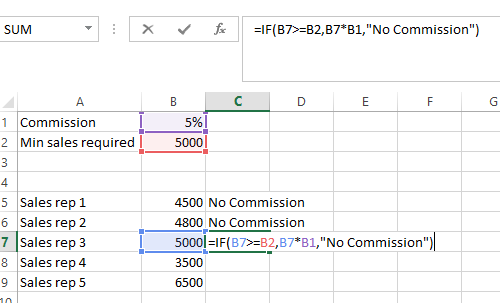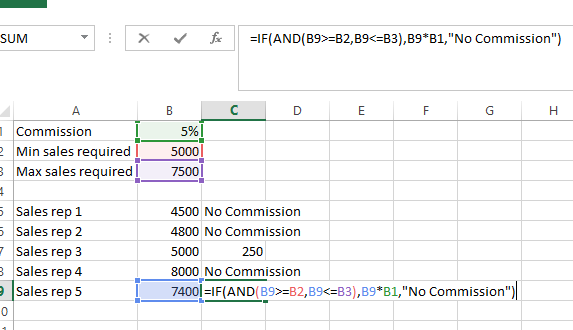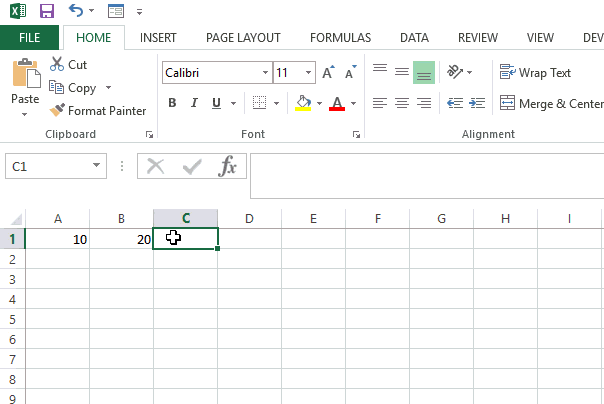New offer! Get 30% off one whole year of Unlimited learning. Subscribe for just £249.99 £174.99. New subscribers only T&Cs apply

•# Logical tests, Comparative Operators and IF statements

Comparative operators are the bare bones of logical tests and logical tests are the bare bones of IF statements. Learn about each in this article.Logical tests give a True or False statement and these can be calculated with the use of comparative operators.  A logical test can be a comparison between two cell references such as A2>B1. Or A logical test can be a comparison between a cell reference and a fixed amount such as C7>= 100.

A comparative operator will always return a True or False result. Excel recognized True and False Statements as 1 and 0. These can be used in further calculations. Because of this, Logical functions are also known as Boolean functions.

There are six comparison operators that can be used in the logical test.  Each of these is shown below:

Equals ( = )

Less than ( < )

Less than or equal to ( < = )

Greater than ( > )

Greater than or equal to ( > = )

Not equal to ( < > )

Logical tests and comparative operators are often used within IF Statements. They can also be combined with AND and OR functions.

AND and OR are also logical operators. On their own they are fairly useless, however, combined with the IF statement, the AND and OR Functions can help create more complex calculations.

With an AND statement, all conditions must be met to get a true result. Whereas with the OR statement only one condition must be met to get a true result.

The syntax for AND is = AND(Logical test 1, logical test 2, logical test 3…) The syntax for OR is =OR(Logical test 1, logical test 2, logical test 3…)

### What is the use of Comparative Operators, logical tests, and IF statements?

The IF function is a powerful function. It allows users to replace the True or False statement with a value or text of choice. The IF function can be combined with the AND or OR functions, along with many other functions such as the Lookup functions.

The best way to understand the use of logical tests and IF statements are by way of an example. Suppose an accountant needs to calculate commission at 5% for each sales staff if they reach a sales target of £5000. And no commission if it is not reached.

We can use logical tests to see if the conditions are met for the commission. If the conditions are met, we can use IF to replace the true and false values with the commission calculation.Looking at the following IF statement (which can be seen in the image above)

IF(B7>=B2, B7*B1,” No Commission”)

In English, this can be read as:

If the content in cell B7 (5000) is greater or equal to the content in cell B2 (5000), then take the value of B7 (5000) and multiply it by the value of cell B1 (5%). If the value of the content in cell B7 is not greater than B2 not, then return the text ‘No Commission’.

Let us explore a second example. This time sales between £5000 and £7500 are eligible for commission. Anything outside of this, 0 commission is paid on the total sales. The accountant must now calculate the commission for each sales rep.Looking at the IF statement:

IF(AND(B9>=B2,B9<=B3),B9*B1,”No Commission”)

IF the content from the cell B9 is greater or equal to the content of cell B2 AND the content of cell B2 is less than or equal to the content of cell B2, then take the content from cell B9 and multiply it by the content in cell B1 otherwise use the text ‘No Commission’

### How to construct an IF statementThe syntax for an IF statement is.

=IF (Logical Test, [Value if True], [Value if False]).

Basic IF statements, IF statements with conditional AND’s or OR’s, and Nested IF statements are all based around this same syntax.

We want to construct an IF statement that will return the text ‘Lower’ if the value of cell A1 is lower than the value of cell B1. And return the text ‘Higher’ if the value is higher.

Step 1: Select the cell in which you want your formula and enter =IF(

Step 2: Identify the logical test. We want to know if the content of cell A1 (10) is lower than, so we would use the < operator, the content of cell B1 =IF(A1<B1,

Step 3: Enter the value to be displayed when true. As we are looking to enter a text value when true, we would place the text in quotes. This is then followed by a comma. =IF(A1<B1,”Lower”,

Step 4: Enter the value to be displayed when false. Again, as we are using text we would display that value in quotes, and we would complete the formula by closing the bracket. =IF(A1<B2,”Lower”, “Higher”)